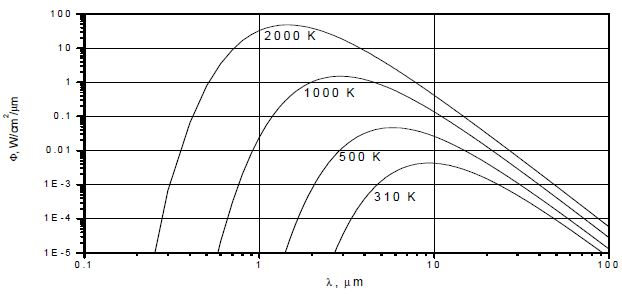Electromagnetic waves have been emitted by all solid bodies having temperatures higher than absolute zero (-273°C). Infrared radiation is the thermal radiation part of this energy.

## Wien’s Displacement Law

The correlation between the temperature and emitting peak wavelength of a solid body is described by Wien’s Displacement Law as follows:

 λmax= 2898/T Equation 1 or T= 2898/λmax Equation 2

Where T is the object temperature in K and λ max is the peak wavelength in µm.

Wien’s Displacement Law can be used to determine an object’s specific peak wavelength at a given temperature. For a solid body, the intensity curve of all emitted wavelengths is relatively broad in accordance with Max Planck.

The intensity curves of radiated energy radiated energy versus wavelength for different temperatures of an ideal black body radiator are presented in Figure 1.Figure 1. Radiated Energy versus wavelength

The energy emitted by a hot object at a temperature of 1000K is much higher than that of an object at 500K. For both temperatures, some amount of energy is in the infrared, near infrared, and visible regions. As shown in Figure 1, shifting of the peak radiated energy curve towards lower wavelengths and the visible region can be observed for the 1000K object.

This is in good agreement with the fact that a 1000K object glows and can be sensed radiating from the surface. Conversely, the object at 500K does not glow as it emits energy in the form of infrared radiation, but still can be sensed. From the peak radiated energy vs. target temperature curve for an 8-14µm band pass window, the following values can be obtained using Equation 2:

T8µm = 2898/8 = 362K = 89°C = 192.6°F

T14µm = 2898/14 = 207K = -66°C = -86.9°F

From these results, one can observe that an 8-14µm band pass window covers peak radiated energy for object temperatures between –86.9°F to 192.6°F. The following table summarizes the calculated peak radiated energy (λmax) for select object temperatures by applying Equation 1:

Table 1. Peak radiated energy (λmax) for select object temperatures

Object Temperature λmax
2000K 1727°C 3140.6°F 1.45µm
1000K 727°C 1340.6°F 2.90 µm
500K 227°C 440.6°F 5.80 µm
310K 37°C 98.6°F 9.35 µm
300K 27°C 80.6°F 9.66 µm
294.1K 21.1°C 70°F 9.86 µm

## Conclusion

The results conclude that the object temperature range has to be taken into account in the selection of an optical window/filter for thermopile detectors to ensure the transmission of the peak radiated energy.This information has been sourced, reviewed and adapted from materials provided by Dexter Research.

## Citations

• APA

Dexter Research Center, Inc.. (2019, December 24). An Introduction to Infrared Radiation and Radiometry. AZoSensors. Retrieved on November 28, 2020 from https://www.azosensors.com/article.aspx?ArticleID=590.

• MLA

Dexter Research Center, Inc.. "An Introduction to Infrared Radiation and Radiometry". AZoSensors. 28 November 2020. <https://www.azosensors.com/article.aspx?ArticleID=590>.

• Chicago

Dexter Research Center, Inc.. "An Introduction to Infrared Radiation and Radiometry". AZoSensors. https://www.azosensors.com/article.aspx?ArticleID=590. (accessed November 28, 2020).

• Harvard

Dexter Research Center, Inc.. 2019. An Introduction to Infrared Radiation and Radiometry. AZoSensors, viewed 28 November 2020, https://www.azosensors.com/article.aspx?ArticleID=590.